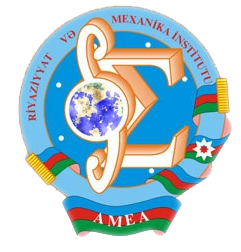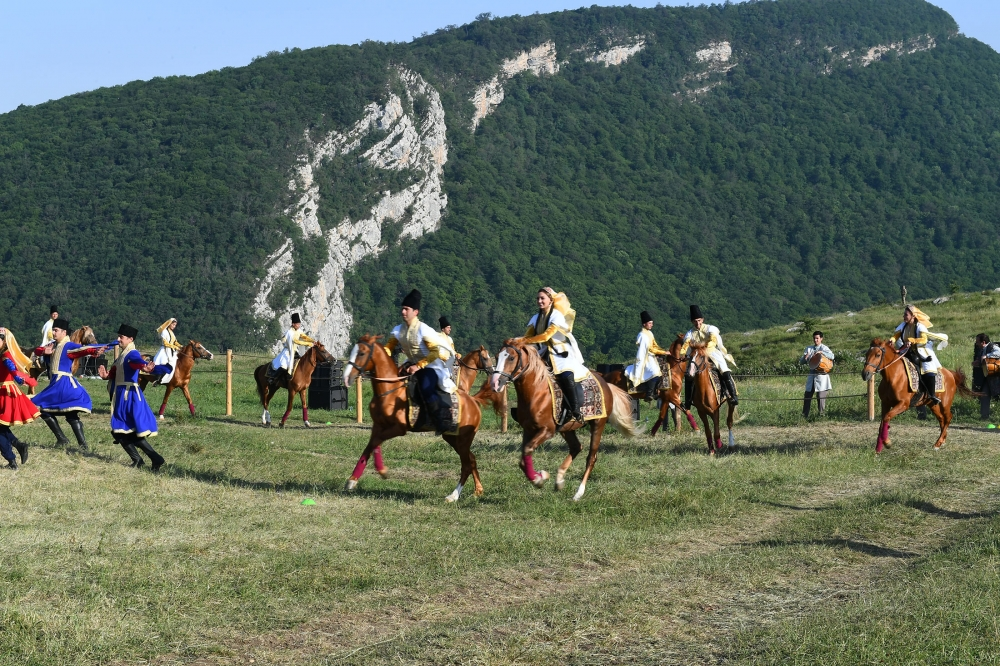Riyaziyyat və Mexanika İnstitutu

## Qarabağ xəbərləri### Şuşada Heydər Əliyev Fondunun təşkilatçılığı ilə “Musiqi irsi və Qarabağ atları Cıdır düzündə” adlı kompozisiya təqdim olunub## Transactions 2010/4/XXX

 Year: 2010 Volume: 4 Number: XXX Aliev B.A. Abstract PDF Solvability of a boundary value problem for a second order elliptic-differential operator equation with spectral parameter in the equation and boundary conditions [PDF] 3 Aliyev S.S. Abstract [PDF] Weighted Morrey a priori estimates for Poisson equation [PDF] 17 Babayeva S.F. Abstract [PDF] On regular solvability of a boundary value problem with operator boundary condition [PDF] 25 Bagirov Sh.G. Abstract [PDF] On asymptotic properties of solutions to nonlinear elliptic equation [PDF] 35 Bandaliev R.A. Abstract [PDF] On a two-weight criterion for Hardy type operator in weighted variable Lebesgue spaces with measures [PDF] 45 Garayev T.Z. Abstract [PDF] “Kadets (1=4) – theorem” and multipliers of type (p; p) [PDF] 55 Huseynov H.M., Jamshidipour A.H. Abstract [PDF] On Jost solutions of Sturm-Liouville equations with spectral parameter in discontinuity condition [PDF] 61 Ibadova I.A. Abstract [PDF] A model of motion of two interacting particles [PDF] 69 Ilyasov N.A. Abstract [PDF] Estimations of the smoothness modules of convolution of two periodic functions by means of their best approximations in Lp(T) (the case of different metrics) [PDF] 75 Ismailov Z., Erol M. Abstract [PDF] The spectrum structure of normal operators [PDF] 89 Kuliyev H.F., Jabbarova K.Sh. Abstract [PDF] Optimal control problem with control at the high coeffcients for the nonlinear hyperbolic equation without uniqueness theorem of solution of initial-boundary value problem [PDF] 97 Manafov M.Dzh. Abstract [PDF] On floquet solutions for periodic “weight” equation with distributions [PDF] 105 Mansimov K.B., Amirova R.R. Abstract [PDF] Necessary oprimality contions in a control problem described by a system of Volterra type two-diemnsional diffrence eqautions [PDF] 111 Mukhtarov F.Sh., Mukhtarov O.Sh., Kadakal M. Abstract [PDF] Fold completeness for discontinuous boundary value problem with spectral parameter in the boundary and transmissions condition [PDF] 123 Muradov T.R., Farahani S. Abstract [PDF] On basisity of some perturbed system of exponents in Lp [PDF] 129 Najafov T.I. Abstract [PDF] nz-plane, nz-analytic functions [PDF] 135 Parolya N.R., Yeleyko Y.I. Abstract [PDF] Killed Markov decision processes on finite time interval for countable models [PDF] 141 Pashayev N.J. Abstract [PDF] On an inverse problem for a reaction-diffusion type system [PDF] 153 Sabzaliyev M.M. Abstract [PDF] On asymptotics of solution to a boundary value problem for a quasilinear hyperbolic equation in an infinite strip [PDF] 159 Yusufov V.Sh. Abstract [PDF] Semigroups of homeomorphisms and dimension [PDF] 171
Azərbaycanda COVID-19 ilə bağlı statistika
• Virusa yoluxan

599713

• Sağalan

569238

• Yeni yoluxan

1210

• Aktiv xəstə

22456

• Ölüm halı

8019

• Test edilib

5,633,054